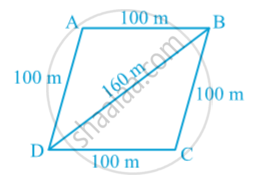# Application of Heron’s Formula in Finding Areas of Quadrilaterals

#### text

Examples: Sanya has a piece of land which is in the shape of a rhombus (see following Fig). She wants her one daughter and one son to work on the land and produce different crops. She divided the land in two equal parts. If the perimeter of the land is 400 m and one of the diagonals is 160 m, how much area each of them will get for their crops?Solution : Let ABCD be the field.
Perimeter = 400 m
So, each side = 400 m ÷ 4 = 100 m.
i.e. AB = AD = 100 m.
Let diagonal    BD = 160 m.
Then semi-perimeter s of ∆ ABD is given by

s = (100 + 100 + 160)/2 m = 180 m

Therefore, area of ∆ ABD

=sqrt (180 (180 - 100)(180 - 100)(180 - 160))

= sqrt (180 * 80 * 80 * 20)   m^2 = 4800 m^2

If you would like to contribute notes or other learning material, please submit them using the button below.

### Shaalaa.com

Application of Heron’s Formula in Finding Areas of Quadrilaterals. [00:11:36]
S
0%